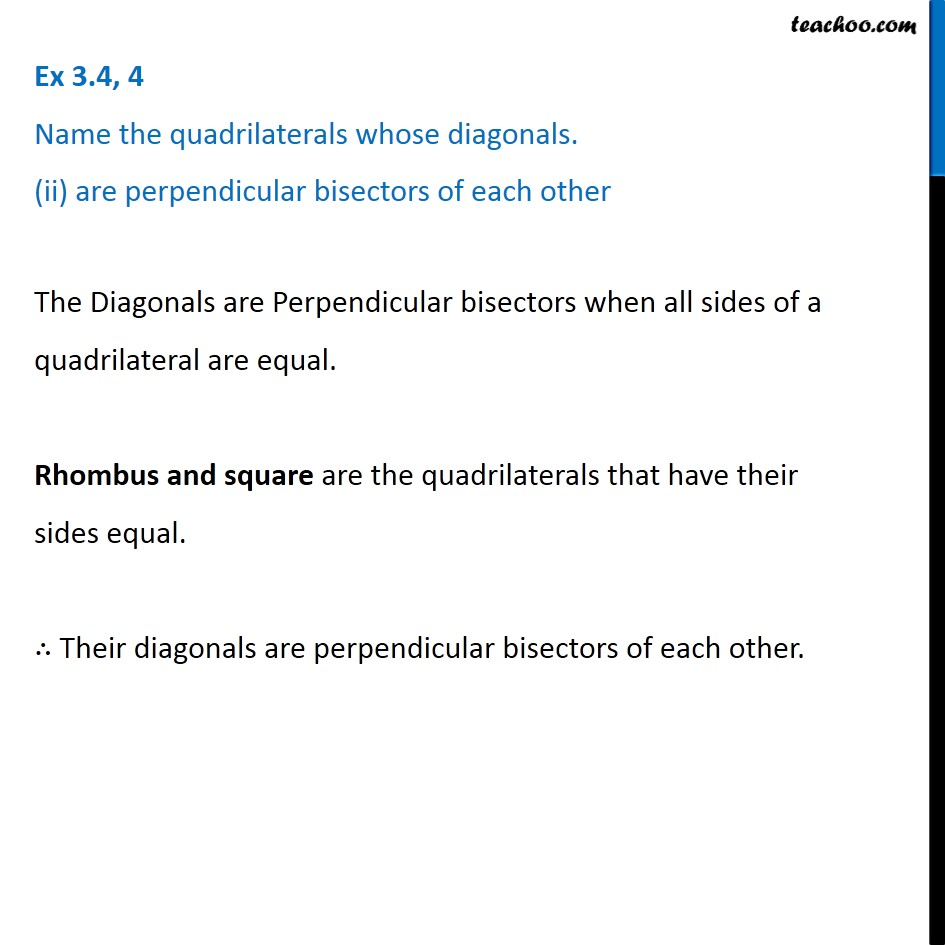1. Chapter 3 Class 8 Understanding Quadrilaterals
2. Serial order wise
3. Ex 3.4

Transcript

Ex 3.4, 4 Name the quadrilaterals whose diagonals. (ii) are perpendicular bisectors of each other The Diagonals are Perpendicular bisectors when all sides of a quadrilateral are equal. Rhombus and square are the quadrilaterals that have their sides equal. ∴ Their diagonals are perpendicular bisectors of each other.